# Bihar Board Class 9th Maths Solutions Chapter 11 Constructions Ex 11.1

Bihar Board Class 9th Maths Solutions Chapter 11 Constructions Ex 11.1 Textbook Questions and Answers.

## BSEB Bihar Board Class 9th Maths Solutions Chapter 11 Constructions Ex 11.1Question 1.
Construct an angle of 90° at the initial point of a given ray and justify the construction.
Solution:
Steps of Construction :

1. Draw a ray OA.
2. With its initial point O as centre and any radius, draw an arc CDE, cutting OA at C.3. With centre C and same radius (as in step 2), draw an arc, cutting the arc CDE at D.
4. With D as centre and the same radius, draw an arc cutting the arc CDE at E.
5. With D and E as centres, and any. convenient radius (more than $$\frac { 1 }{ 2 }$$ DE ), draw two arcs intersecting at P.
6. Join OP. Then ∠AOP = 90°.

Justification :
By construction, OC = CD = OD
∴ ∆ OCD is an equilateral triangle. So, ∠COD = 60°.
Again, OD = DE = EO
∴ ∆ ODE is also an equilateral triangle. So, ∠DOE = 60°.
Since OP bisects ∠DOE, so ∠POD = 30°.
Now, ∠AOP = ∠COD + ∠DOP = 60° + 30° = 90°.Question 2.
Construct an angle of 45° at the initial point of a given ray and justify the construction.
Solution:
Steps of Construction

1. Draw a ray OA.
2. With O as centre and any suitable radius draw an arc cutting OA at B.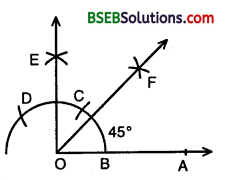3. With B as centre and same radius cut the previous drawn arc at C and then with C as centre and same radius cut the arc at D.
4. With C as centre and radius more than half CD draw an arc.
5. With D as centre and same radius draw another arc to ut the previous arc at E.
6. Join OE. Then ∠AOE = 90°.
7. Draw the bisector OF of ∠AOE. Then ∠AOF = 45°.

Justification :
By construction, ∠AOE = 90° and OF is the bisector of ∠AOE
∴ ∠AOF = $$\frac { 1 }{ 2 }$$∠AOE = $$\frac { 1 }{ 2 }$$ x 90° = 45°.Question 3.
Construct the angles of the following measurements :
(i) 30°
(ii) 22$$\frac { 1° }{ 2 }$$
(iii) 15°.
Solution:
(i) Steps of Construction :

• Draw a ray OA.
• With its initial point O as centre and anyx radius, draw an arc, cutting OA at C.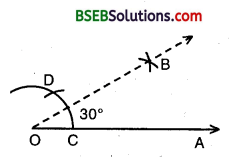• With centre C and same radius (as in step 2). Draw an arc, cutting the arc of step 2 in D.
• With C and D as centres, and any convenient radius (more than $$\frac { 1 }{ 2 }$$ CD), draw two arcs intersecting at B.
• Join OB. Then ∠AOB = 30°.

(ii) Steps of Construction :

• Draw an angle AOB = 90°.
• Draw the bisector OC of ∠AOB, then ∠AOC = 45°.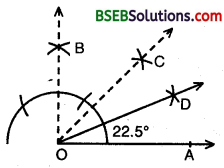• Bisect ∠AOC, such that ∠AOD = ∠COD = 22.5°.
Thus, ∠AOD = 22.5°.

(iii) Steps of Construction :

• Construct an ∠AOB = 60°.
• Bisect ∠AOB so that ∠AOC = ∠BOC = 30°.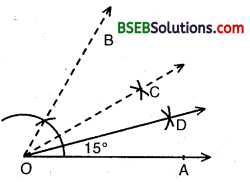• Bisect ∠AOC, so that ∠AOD = ∠COD = 15°.
Thus, ∠AOD = 15°.Question 4.
Construct the following angles and verify by measuring them by a protractor :
(i) 75°
(ii) 105°
(iii) 135°
Solution:
(i) Steps of Construction :

• Draw a ray OA.
• Construct ∠AOB = 60°.
• Construct ∠AOP = 90°.
• Bisect ∠BOP so that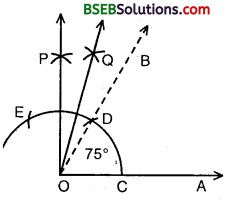• So, we obtain ∠AOQ = ∠AOP + ∠BOQ = 60° + 15° = 75°.

Verification :
On measuring ∠AOQ, with the protractor, we find ∠AOQ – 75°.

(ii) Steps of Construction :

• Draw a line segment XY.
• Construct ∠XYT = 120° and ∠XYS = 90°, so that ∠SYT = ∠XYT – ∠XYS = 120° – 90° = 30°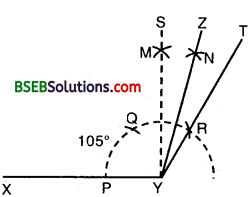• Bisect angle SYT, by drawing its bisector YZ.
• Then ∠XYZ is the required angle of 105°.

(iii) Steps of Construction :

• Draw ∠AOE = 90°. Then, ∠LOE = 90°.• Draw the bisector OF of ∠LOE.
• Then, ∠AOF = 135°.Question 5.
Construct an equilateral triangle, given its side and justify the construction.
Solution:
Let us draw an equilateral triangle of side 4.6 cm (say).
Steps of Construction :

1. Draw BC = 4.6 cm.
2. With B and C as centres and radii equal to BC = 4.6 cm, draw two arcs on the same side of BC, intersecting each- other at A.3. Join AB and AC.
4. Then, ABC is the required equilateral triangle.

Justification :
Since by construction :
AB = BC = CA = 4.6 cm.
∴ ∆ ABC is an equilateral triangle.